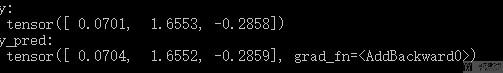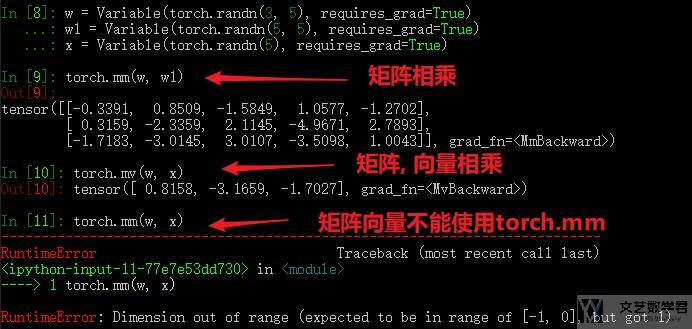# 使用Pytorch解决普通优化问题2019年12月15日07:03:47

1293字阅读4分18秒

## 简介

Pytorch可以对神经网络进行梯度下降并优化网络的参数. 但同时, 对于普通的优化问题, 我们也可以使用Pytorch来进行解决. 即我们也可以利用梯度下降的思路来寻求最优解.

## Pytorch解决普通优化问题

1. import torch
2. import torch.optim as optim
4. w = Variable(torch.randn(3, 5), requires_grad=True# 这里变量的定义需要注意, 是需要梯度的
6. x = Variable(torch.randn(5))
7. y = Variable(torch.randn(3))
8. optimizer = optim.SGD([w,b], lr=0.01) # 我们要优化的是w和b两个参数
9. num_epochs = 100
10. for epoch in range(num_epochs):
12.     y_pred = torch.mv(w, x) + b # torch.mv表示矩阵与向量相乘
13.     loss = ((y_pred-y)**2).sum()
14.     loss.backward()
15.     optimizer.step()
16.     if epoch % 5 == 0:
17.         print(loss)
18. y_pred = torch.mv(w, x) + b
19. print('y: \n', y)
20. print('y_pred: \n', y_pred)## 一些其他操作

### 矩阵转置

1. pt_matrix_ex # 原始矩阵
2. pt_matrix_ex.t() # 转置后的矩阵

### 矩阵相乘

1. torch.mm(P, Q.t())

### 矩阵与向量相乘• 微信公众号
• 关注微信公众号
•• QQ群
• 我们的QQ群号
•• 本文由 发表于 2019年12月15日07:03:47
• 转载请务必保留本文链接：https://mathpretty.com/11519.html